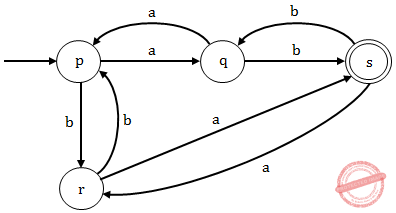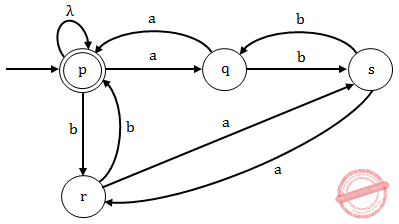## UGC NET CS 2014 June-paper-2

 Question 1
Infrared signals can be used for short range communication in a closed area using _______ propagation.
 A ground B sky C line of sight D space
Data-Communication       FM-Signal
Question 1 Explanation:
Propagation methods are 3 types
1. Ground
2. Sky
3. Line of sightQuestion 2
 A Physical B Network C Datalink D Application
Computer-Networks       Hardware-and-various-devices-in-networking
Question 2 Explanation:
 Question 3
The minimum frame length for 10 Mbps Ethernet is _______ bytes and maximum is _______ bytes.
 A 64 & 128 B 128 & 1518 C 1518 & 3036 D 64 & 1518
Computer-Networks       Ethernet
Question 3 Explanation:
10Base-T is the Ethernet wiring standard for 10 Mbps (megabits per second) for a maximum distance of approximately 100 meters per segment over unshielded twisted pair cables terminated with RJ-45 connectors.Note: 100Base-T (Fast Ethernet) and 100Base-T (Gigabit Ethernet)
 Question 4
The bit rate of a signal is 3000 bps. If each signal unit carries 6 bits, the baud rate of the signal is _______.
 A 500 baud/sec B 1000 baud/sec C 3000 baud/sec D 18000 baud/sec.
Computer-Networks       Baud-Rate
Question 4 Explanation:
→ Bit rate is nothing but number of bits transmitted per second
→ Baud rate is nothing but number of signals units transmitted per unit time.
Given data,
-- Bit rate of a signal=3000 bps
-- Each signal unit carries=6 bits
-- Baud rate=?
Step-1: Baud rate= Bit rate of signal / baud rate
= 3000/6
= 500 baud/sec
 Question 5
Match the following :A a-iv, b-v, c-ii, d-iii, e-i B a-v, b-iv, c-i, d-ii, e-iii C a-i, b-iii, c-ii, d-v, e-iv D a-i, b-ii, c-iv, d-iii, e-v
Computer-Networks       TCP/IP-Layers
Question 5 Explanation:
Physical layer→ Transmission of bit stream
Data link layer→ Formation of frames
Network layer→ Move packets from one destination to other
Transport layer→ Process to process message delivery
Application Layer→ Allow resources to network access
 Question 6
A grammar G is LL(1) if and only if the following conditions hold for two distinct productions A → α | β
1. First (α) ∩ First (β) ≠ {a} where a is some terminal symbol of the grammar.
2. First (α) ∩ First (β) ≠ λ
III. First (α) ∩ Follow (A) = φ if λ ∈ First (β)
 A I and II B I and III C II and III D I, II and III
Compiler-Design       Parsers
Question 6 Explanation:
A grammar G is LL(1) if and only if the following conditions hold for two distinct productions:
A → α | β
1. First (α) and First (β) must be disjoint if none of α and β contains NULL move.
2. At most one of the strings α or β can drive NULL move i.e. α → NULL(since First (α) and First (β) are disjoint). In this case, First (β) and Follow(A) must be disjoint.
 Question 7
Which of the following suffices to convert an arbitrary CFG to an LL(1) grammar ?
 A Removing left recursion alone B Removing the grammar alone C Removing left recursion and factoring the grammar D None of the above
Compiler-Design       Parsers
Question 7 Explanation:
→ Left recursion removing (or) factoring the given grammar are not sufficient to convert an arbitrary CFG to an LL(1) grammar.
→ To convert an arbitrary CFG to an LL(1) grammar we need to remove the left recursion and as well as left factoring without that we cannot convert.
 Question 8
A shift reduce parser suffers from
 A shift reduce conflict only B reduce reduce conflict only C both shift reduce conflict and reduce reduce conflict D shift handle and reduce handle conflicts
Compiler-Design       Parsers
Question 8 Explanation:
→ A shift-reduce parser scans and parses the input text in one forward pass over the text, without backing up. (That forward direction is generally left-to-right within a line, and top-to-bottom for multi-line inputs.) The parser builds up the parse tree incrementally, bottom up, and left to right, without guessing or backtracking.
A shift-reduce parser works by doing some combination of Shift steps and Reduce steps, hence the name.
→ A Shift step advances in the input stream by one symbol. That shifted symbol becomes a new single-node parse tree.
→ A Reduce step applies a completed grammar rule to some of the recent parse trees, joining them together as one tree with a new root symbol.
*** A shift reduce parser suffers from both shift reduce conflict and reduce reduce conflict.
 Question 9
The context free grammar for the language L = {anbmck | k = |n – m|, n ≥ 0, m ≥ 0, k ≥ 0} is
 A S → S1S3, S1 → aS1c | S2| λ, S2 → aS2b|λ, S3 → aS3b| S4 | λ, S4 → bS4c|λ B S → S1S3, S1→ aS1S2c | λ, S2 → aS2b|λ, S3 → aS3b| S4 |λ, S4 → bS4c|λ C S → S1|S2, S1→ aS1S2c | λ, S2 → aS2b | λ, S3 → aS3b | S4 |λ, S4 → bS4c|λ D S → S1 | S3, S1→ aS1c|S2 | λ, S2 → aS2b | λ, S3 → a S3b| S4 | λ, S4 → bS4c | λ
Theory-of-Computation       Context-Free-Grammar
Question 9 Explanation:
L = { λ, ab, ac, bc, aabb,aabc,aac, bbc, ........}
Option(A): Option(A) will generate the string "acab" which does not belongs to the sequence anbmck . So, it is not the context free grammar for the language L.
Option(B): Option(B) will generate the string "acab" which does not belongs to the sequence anbmck. So, it is not the context free grammar for the language L. Option(C): In this option production S3 and S4 are unreachable so it is not the context free grammar for the language L. Option (D): The grammar given in this option can generate { λ, ab, ac, bc, aabb,aabc,aac, bbc, ........}. So it is the context free grammar for the language L.
 Question 10
The regular grammar for the language
L = {w|na(w) and nb(w) are both even,
w ∈ {a, b}*} is given by :
(Assume, p, q, r and s are states)
 A p → aq | br | λ, q → bs | ap r → as | bp, s → ar | bq, p and s are initial and final states. B p → aq | br, q → bs | ap r → as | bp, s → ar | bq, p and s are initial and final states. C p → aq | br | λ, q → bs | ap r → as | bp, s → ar | bq p is both initial and final states. D p → aq | br, q → bs | ap r → as | bp, s → ar | bq p is both initial and final states
Theory-of-Computation       Regular-Language
Question 10 Explanation:
L={ λ. ab, λab, λaabb, aabb, ..........}
Option (A): The grammar given in this option does not contain a state which is accepting a terminal "λ" , having zero number of "a" and "b". Since the grammar is not accepting λ so it not the regular grammar for the given language L.Option (B): The grammar given in this option is not generating any string "λ" which is having zero number of "a" and "b". Since the grammar is not generating λ so it not the regular grammar for the given language L.Option (C): Here state "p" is the initial and final state and the grammar given here is generating string "λ" , having zero number of "a" and "b". Since the grammar is accepting λ so it is the regular grammar for the given language L.Option (D): In this option (p) is the initial and final state but the grammar is not generating any string "λ" which also belongs to the given language L. So this grammar is not the regular grammar for the given language L.Question 11
KPA in CMM stands for
 A Key Process Area B Key Product Area C Key Principal Area D Key Performance Area
Software-Engineering       CMMI
Question 11 Explanation:
KPA(Key process area) in Capability Maturity Model(CMM):
A Process Area is a cluster of related practices in an area that, when implemented collectively, satisfy a set of goals considered important for making significant improvement in that area. All CMMI process areas are common to both continuous and staged representations.
 Question 12
Which one of the following is not a risk management technique for managing the risk due to unrealistic schedules and budgets ?
 A Detailed multi source cost and schedule estimation. B Design cost C Incremental development D Information hiding
Software-Engineering       Software-testing
Question 12 Explanation:
Information hiding is not a risk management technique for managing the risk due to unrealistic schedules and budgets.
There are 12 questions to complete.

Register Now